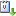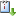Usted está aquí: Soliton dynamics for the generalized choquard equation

# Soliton dynamics for the generalized choquard equation

Marco Ghimenti (Universidad de Pisa) - Jueves 14 de Noviembre de 2013, 11:00 hrs.
Ponente:
Cuándo 14/11/2013 de 11:00 a 12:00 Graciela SalicrupvCaliCal

We study the soliton dynamics for a class of nonlinear Schrödinger equations with a non-local nonlinear term. We prove that soliton solutions under the effect of an external potential exhibit in the semi-classical limit a particle-like behavior. We consider what we call generalized Choquard equation where the nonlinear term is
$f(u)=(|x|^{(\theta -N )} * |u|^p)|u|^{(p−2)}u.$
If $$(\theta -N)=1$$ and $$p=2$$ the problem is very well studied, and there exists a precise description of the soliton dynamics, and the proof relies on the non degeneracy of ground states.

In the general case the ground state solutions are not known to be unique or non-degenerate.
This problem can be avoided when the nonlinear effect is sufficiently strong, and we developped a method that in this case provide a description of soliton dynamics